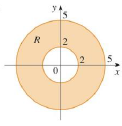Chapter 15.3, Problem 1E

Chapter
Section
Textbook Problem

A region R is shown. Decide whether to use polar coordinates or rectangular coordinates and write ∬ R f ( x ,   y )   d A as an iterated integral, where f is an arbitrary continuous function on R.1.To determine

To write: An iterated integral by deciding whether to use polar coordinates or rectangular coordinates.

Explanation

Formula used:

If f is a polar rectangle R given by 0arb,αθβ, where 0βα2π , then, Rf(x,y)dA=αβabf(rcosθ,rsinθ)rdrdθ (1)

Calculation:

Since the given region is the circular annulus, it is easier to use polar coordinates.

From the figure given in the problem, it is observed that radius r ranges from 2 to 5 and θ varies from 0to 2π .

Therefore, the polar coordinates is, R={(r,θ)|2r5,0θ2π}

Still sussing out bartleby?

Check out a sample textbook solution.

See a sample solution

The Solution to Your Study Problems

Bartleby provides explanations to thousands of textbook problems written by our experts, many with advanced degrees!

Get Started

Radical Expressions Simplify the expression. 50. (a) 125+45 (b) 543163

Precalculus: Mathematics for Calculus (Standalone Book)

Expand each expression in Exercises 122. (x+1x)2

Finite Mathematics and Applied Calculus (MindTap Course List)

In Exercises 33-38, rewrite the expression using positive exponents only. 37. 120(s + t)3

Applied Calculus for the Managerial, Life, and Social Sciences: A Brief Approach

In Problems 15-28, perform the operations if possible. 23. .

Mathematical Applications for the Management, Life, and Social Sciences

Find the derivative of the function. y=(x+1x)5

Single Variable Calculus: Early Transcendentals

0 1 2 ∞

Study Guide for Stewart's Single Variable Calculus: Early Transcendentals, 8th

True or False:

Study Guide for Stewart's Multivariable Calculus, 8th